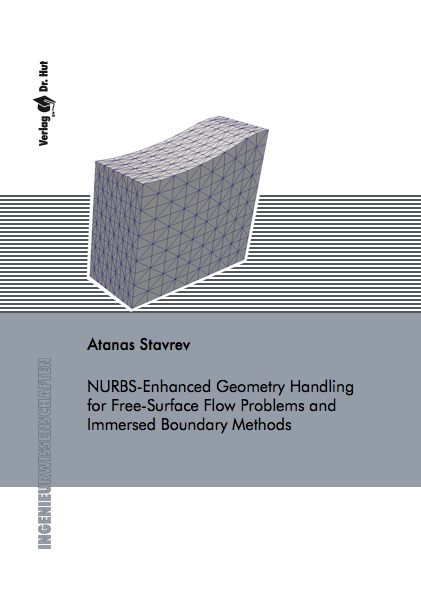Datenbestand vom 21. April 2021aktualisiert am 21. April 2021

# ISBN 978384392722272,00 € inkl. MwSt, zzgl. Versand

Atanas Stavrev
NURBS-Enhanced Geometry Handling for Free-Surface Flow Problems and Immersed Boundary Methods

149 Seiten, Dissertation Rheinisch-Westfälische Technische Hochschule Aachen (2016), Softcover, A5

## Zusammenfassung / Abstract

The thesis at hand addresses the role of geometry representation in modern numerical analysis of engineering problems. Two topics are covered: The accurate computation of free-surface flows and the immersed boundary analysis.

In the scope of free-surface flow simulations, a free-boundary problem is solved, which implies that the geometrical position of parts of the domain of interest is unknown. Therefore, the accuracy of the numerical solution depends strongly on the computation of geometric quantities like normal vectors and curvatures. Consequently, a suitable geometry representation of the unknown boundary is needed, which usually requires a much finer finite element discretization of the computational domain than the flow solution itself. This problem is addressed by the derivation the space-time NURBS-enhanced Finite Element Method (space-time NEFEM), which uses standard functions to discretize the unknown function field in combination with an improved geometry representation. The application of the space-time NEFEM to free-surface flow is investigated and a kinematic rule for the NURBS motion in time is derived. Numerical examples show the ability of the space-time NEFEM to account for both pressure discontinuities and surface tension effects, as well as to compute smooth free-surface forms and improve mass conservation in two- and three-dimensional simulations.

In the context of immersed boundary methods, the geometry resolution in intersected elements which contain portions of both the physical and the fictitious domains is assessed. Accounting for the fact that the immersed objects possess a NURBS boundary description, the NURBS entity is introduced into the geometry mapping for the respective element. In this way a pure NURBS-enhanced geometry mapping is derived. In a second step, an alternative geometry resolution is proposed through approximation of the NURBS boundary with quadratic Lagrange elements. Consequently, suitable quadrature rules are derived, which drastically reduce the required number of quadrature points for accurate geometry integration. The performance of the novel integration strategy is compared to the standard approach for two three-dimensional simulations of linear elasticity problems. Reported are superior computational efficiency and improved convergence behavior. The assessment is concluded by application of the quadratic Lagrange elements to a complex coupled simulation of fracture mechanics of human bones.